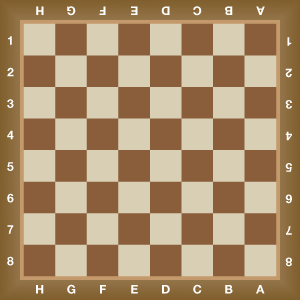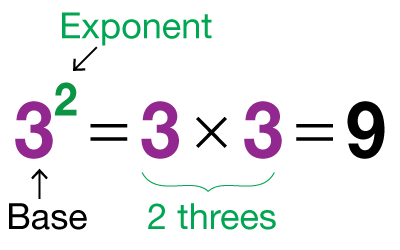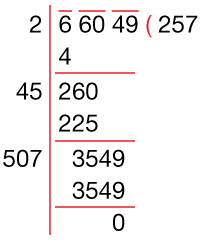# Squares and Square Roots

Go back to  'Exponents, Squares, Cubes'

Introduction: Have you played chess or seen a chessboard? It is a unique arrangement of black and white squares. There are eight squares on one side. If someone asks you how many squares there are on the chessboard, you can count the number and come up with the answer. Or you can multiply 8 by itself to get the square of 8 and say the right answer 64. Let us take another example. Say you have a square of side 5 cm. The area of this square would be the square of 5, which is 25.For both these examples, square roots can also be calculated from both the answers. The number of squares on one side of a chessboard can be found by finding the square root of 64, which is 8, which is equal to the number of squares on one side. Again, if a square of area 25 sq. Cm is given to you, and you can find the value of each side of the square just by finding out the square root of 25, which is 5, which is equal to each side of the square.

## The Big idea: Squares and square roots

### A simple idea: The Most Common Exponent

When a number is multiplied by itself several times, it is said to be raised by its exponent. So, if you multiply the number 3 by itself four times, you would write it as 3 x 3 x 3 x 3 or 34. Here the answer is 81, and the number 4 is referred to as the exponent of 3.The most common and useful exponent is 2, which we discussed in the introduction. Any number raised to the exponent two is called its square. So, 52 is referred to as the square of 5, while 82 is referred to as the square of 8.

When we talk of square roots, then the exponent is expressed as $${1 \over 2}$$. So, the square root of 25 is referred to as \begin{align}25^\frac12.\end{align}

### Methods for Finding Square Roots

We know that a prime number is a number which is divisible only by 1 and by itself. So, 2, 5, 7, 11 are all prime numbers. And we also know about the process of factorisation in which a number is broken up into several numbers which when multiplied together give that number. Let us see the example of how to factorise 42

\begin{align} & 42\,\ =2\times 21 \\ & \ \ \ \ \,\,=2\times \left( 3\times 7 \right) \\ & \ \ \ \ \,\,=2\times 3\times 7 \\ \end{align}

All of the above numbers 2, 3 and seven are referred to as factors of 42, and incidentally, they are all prime factors as well, which means all the factors are prime numbers.

### Prime Factorization Method

When a square number is broken into its prime factors, it helps to find the square root as well. Let us take the example of 144, which is the square of 12.

\begin{align} & 144~=2\times \left( 72 \right) \\ & ~~~~~\ \,~=2\times 2\times \left( 36 \right) \\ & ~~~~~\ \,~=2\times 2\times 2\times \left( 18 \right) \\ & ~~~~~\ \,~=2\times 2\times 2\times 2\times \left( 9 \right) \\ & ~~~~~\ \,~=2\times 2\times 2\times 2\times 3\times 3 \\ & ~~~~~\ \,~=\left( 2\times 2 \right)\times \left( 2\times 2 \right)\times \left( 3\times 3 \right) \\ & ~~~~~\ \,~={{2}^{2}}\times {{2}^{2}}\times {{3}^{2}} \\ & ~~~~~\ \,~={{\left( 2\times 2\times 3 \right)}^{2}} \\ & ~~~~~\ \,~={{\left( 12 \right)}^{2}} \\ \end{align}

Therefore, by breaking up 144 into its prime factors, we can know that it is the square of 12, so its square root would be 12.

### Long Division Method:

This is a more precise way of finding the square root and works even if the number is NOT a perfect square. This is how you go about calculating the square root using the long division method:

Step 1: Group the number whose square root is to be calculated into pairs starting from the units place. These groups are called Periods.

Step 2: Start with the first period from the left and find the largest possible number whose square divides it. This number is your divisor as well as your quotient for this part of the division and ONLY the first period is your dividend.

Step 3: For the next period, the divisor is determined by doubling the current quotient and then annexing it with a suitable digit, which will also act as the quotient for the next period such that the product of the new digit and the quotient is less than or equal to the current period.

Step 4: Repeat the first three step till all the periods have been covered. If the number is a perfect square you won’t need to go into decimals. If in case it is not a perfect square, place a decimal point and start creating periods of TWO ZEROES each for every decimal place in the quotient until the remainder of zero is obtained.## Tips and Tricks

• Use identities to find squares mentally
Remember, \begin{align}(a + b)^2 = a^2 + 2ab + b^2\end{align} and \begin{align}(a - b)^2 = a^2 - 2ab + b^2\end{align}

What’s $$61^2?$$
\begin{align}61^2 = (60 + 1)^2 = 3600 + 120 + 1 = 3721\end{align}

Find $$98^2$$
\begin{align}98^2 = (100 - 2)^2 = 10,000 - 400 + 4 = 9,604\end{align}

• A trick to square numbers that end in 5:

Ignore the 5.
Multiply the rest of the number by its successor.
Attach 25 at the end of this answer and you have your square!

E.g. to find the square to 65:
Ignore 5… we are left with 6.
Multiply 6 by its successor, 7. We get 42.
Attach 25 to the end of this number. So, $$65^2$$ is 4225.

• Trick to guess the approximate square root of a large number, like 75,293

The square root will be a three-digit number as
100 x 100 = 10,000
but 1000 x 1000 = 10,00,000

Jump in 100s.
200 x 200 = 40,000
300 x 300 = 90,000
So, the square root is between 200 and 300
You can stop here or continue...

Now guess a number between 200 and 300… perhaps slightly closer to 300 as 75,293 is closer to the square of 300 than to the square of 200.
270 x 270 = 72,900… just below what we want. Let’s try a higher number:
280 x 280 = 78,400
So, the square root is between 270 and 280
You can stop here or continue...

So now we guess a number between 270 and 280
275 x 275 = 75,625
So, the square root is just less than 275. We can stop here.
Verifying with a calculator we see the square root is 274.4… we came pretty close!

• Trick: Adding consecutive odd numbers gives us perfect squares:

\begin{align}1 + 3 \quad &= 4 \qquad = 2^2\\ 1 + 3 + 5 \quad&= 9 \quad\;\;\;\,= 32\\ 1 + 3 + 5 + 7 \quad &= 16 \quad\;\, = 42\\ 1 + 3 + 5 + 7 + 9 \quad&= 25 \quad\;\,= 52\end{align}
… and so on.